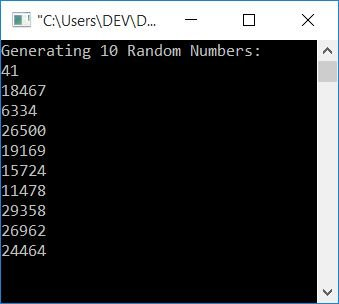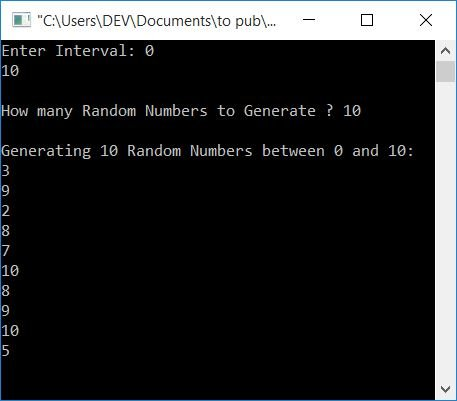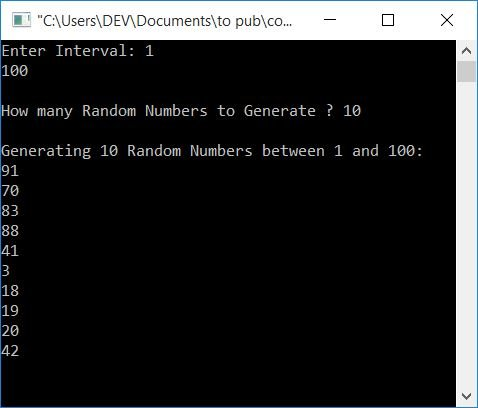# C program to generate random numbers

In this article, you will learn and get code about generating random numbers. The first program generates 10 random numbers. And the second one generates a limited amount of random numbers in a given range. The user will enter the limit and range here at run-time.

## Generate 10 random numbers

This program generates 10 random numbers. The function rand() (from stdlib.h) is used to generate random numbers.

```#include<stdio.h>
#include<conio.h>
#include<stdlib.h>
int main()
{
int i, rnum;
printf("Generating 10 Random Numbers:\n");
for(i=0; i<10; i++)
{
rnum = rand();
printf("%d\n", rnum);
}
getch();
return 0;
}```

This program was built and runs under the Code::Blocks IDE. Here is its sample run:In the above program, the for loop is used to execute the following block of code:

```rnum = rand();
printf("%d\n", rnum);```

10 times. At each execution, a random number using rand() is generated and initialized to the rnum variable. And its value is printed on the output using the printf() function.

## Generate a Range of Random Numbers

Here is another program with an extra feature in comparison to the previous one. It includes a feature that allows the user to define the amount (how many random numbers to generate) and the limit under which random numbers have to be generated.

```#include<stdio.h>
#include<conio.h>
#include<stdlib.h>
#include<time.h>
int main()
{
int i, rnum, amount, start, end;
printf("Enter Interval: ");
scanf("%d%d", &start, &end);
printf("\nHow many Random Numbers to Generate ? ");
scanf("%d", &amount);
printf("\nGenerating %d Random Numbers between %d and %d:\n", amount, start, end);
srand(time(0));
for(i=0; i<amount; i++)
{
rnum = rand()%(end+1-start)+start;
printf("%d\n", rnum);
}
getch();
return 0;
}```

The sample run given below generates 10 random numbers between 0 and 10. This value is entered by the user at runtime.If the function srand() was not used in the above program, then at the second sample run (with the same user input), all the 10 random numbers were the same. Therefore, to generate new random numbers on every sample run, use the srand() function.

And here is another sample run that will generate 10 random numbers between 1 and 100:#### The same program in different languages

C Quiz

« Previous Program Next Program »